# RS Aggarwal Class 10 Solutions Chapter 17 - Perimeter And Areas Of Plane Figures Ex 17A (17.1)

## RS Aggarwal Class 10 Chapter 17 - Perimeter And Areas Of Plane Figures Ex 17A (17.1) Solutions Free PDF

The RS Aggarwal Class 10 Solutions for maths will help you in your exam preparation. The answers provided in the solutions are easy and simple to understand. It will help you if you get stuck at any step of the solution and remember that the solutions are prepared keeping in mind the latest syllabus of CBSE. Scoring good marks will become easy if you prepare for the maths exam from the RS Aggarwal solutions of Class 10.

The solutions are developed by the subject experts comprising all the answers in the well-structured format. With the help of these solutions, you get to know the deeper knowledge of the topics in a simple and understandable language. All the solutions of the RS Aggarwal Class 10 Solutions Chapter 17 Perimeter And Areas Of Plane Figures Ex 17 A are accurate and correctly solved.

## Download PDF of RS Aggarwal Class 10 Solutions Chapter 17– Perimeter And Areas Of Plane Figures Ex 17A (17.1)

Q.1: A footpath of uniform width runs all around the inside of a rectangular field 54 m long and 35 m wide. If the area of the path is 420 $m^{2}$, find the width of the path.

Sol: Area of rectangular field including the foot path = (54 * 35) $m^{2}$

Let the width of the path be x m

Then, area of rectangle plot excluding the path = (54 * 2x) * (35 * 2x)

Area of path = (54 * 35) + (54 * 2x)(35 * 2x)

(54 * 35) + (54 * 2x) (35 * 2x) = 420

1890 – 1890 + 108x + 70x – $4x^{2}$ = 420

178x – $4x^{2}$ = 420

$4x^{2}$ – 178x + 420 = 0

$2x^{2}$ – 89x + 210 = 0

$2x^{2}$ – 84x – 5x + 210 = 0

2x(x – 42) – 5(x – 42) = 0

(x – 42) (2x – 5) = 0

Q.2: The length and the breadth of a rectangular garden are in the 9:5. A 3.5m wide, running all around inside it has an area of 1911 $m^{2}$. Find the dimensions of the garden.

Sol: Let the length and breadth of a rectangular garden be 9x and 5x.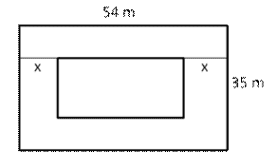Then, area of garden = (9x * 5x) $m^{2}$ = 45 $x^{2}m^{2}$

Length of park excluding the path = (9x – 7) m

Breadth of the park excluding the path = (5x – 7) m

Area of the park excluding the path = (9x – 7)(5x – 7)

Area of the path = $45x^{2}-(9x-7)(5x-7)$

= $45x^{2}-45x^{2}+63x+35x-49$ = 63x + 35x – 49 = (98x – 49) $m^{2}$

(98x – 49) = 1911

98x = 1911 + 49

i.e. 98x = 1960 i.e. x = $\frac{1960}{98}=20m$

Length = 9x = 9 * 20 = 180m

Breadth = 5x = 2 * 20 = 100m

Hence, Length = 180 m and the breadth = 100m

Q.3: A room 4.9 m long and 3.5 m broad is covered with carpet, leaving an uncovered margin of 25 cm all around the room. If the breadth of the carpet is 80 cm, find its cost at Rs. 80 per meter.

Sol: Area of carpet = (4.9 – 0.5)(3.5 – 0.5) $m^{2}$ = 4.4 * 3.0 = 13.2 $m^{2}$

Length of the carpet = $\frac{13.2}{0.80}m$ = 16.5m

Cost of carpet = Rs. 40 per metre

Cost of 16.5 m carpet = Rs. (40 * 16.5) = Rs. 660

Q.4: A carpet is laid on the floor of a room 8m by 5m. There is a border of constant width all around width all around the carpet. If the breadth of the carpet is 80cm, find its cost at Rs. 80 per meter.

Sol: Let the width of the carpet = x meter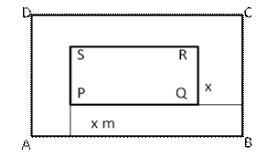Area of floor ABCD = (8 * 5) $m^{2}$

Area of floor PQ.RS without border = (8 – 2x) (5 – 2x) = 40 – 26x + $4x^{2}$

Area of border = Area of floor ABCD – Area of floor PQ.RS

= [40 – (40 – 26x + $4x^{2}$ )] $m^{2}$

= [40 – 40 + 26x – $4x^{2}$ ] $m^{2}$

= (26x – $4x^{2}$) $m^{2}$

Therefore, (26x – $4x^{2}$ ) = 12

i.e 26x – $4x^{2}$ – 12 = 0

i.e $-4x^{2}$ + 26x -12 = 0

i.e $2x^{2}$ – 13x + 6 = 0

i.e $2x^{2}$ – 12x – x – 6 = 0

i.e 2x(x – 6) – 1 (x – 6) = 0

i.e (2x – 1) (x – 6) = 0

i.e $x=\frac{1}{2}$ or x = 6

Width = $\frac{1}{2}m$ = 0.5m[Neglect x = 6 because width is not more than breadth]

Width = (0.5 * 100) cm = 50cm

Q.5: A 80m by 64 m rectangular lawn has two roads, each 5m wide, running through its middle, one parallel to its length and the other parallel to its breadth. Find the cost of gravelling the roads at Rs. 40 per $m^{2}$.

= (80 * 5) $m^{2}$ = 400 $m^{2}$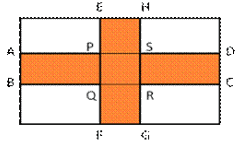= (64 * 5) $m^{2}$ = 320 $m^{2}$

Area of common road PQRS = (5 * 5) $m^{2}$ = 25 $m^{2}$

Area of the road to be graveled = (400 + 320 – 25) $m^{2}$ = 695 $m^{2}$

Cost of gravelling the roads = Rs. (695 * 24) $m^{2}$ = 16680

Q.6: The dimensions of a room are 14m * 10m * 6.5m. There are two doors and the 4 windows in the room. Each door measures 2.5m * 1.2m and each window measures 1.5m * 1m. Find the cost of painting the four walls of the room at Rs. 35 per $m^{2}$

Sol: Area of four walls of room = 2(l + b) * h = 2(14 + 10) * 6.5 = 2 * 24 * 6.5 = 312 $m^{2}$

Area of two doors = 2 * (2.5 * 1.2) $m^{2}$ = 6 $m^{2}$

Area of four windows = 4 (1.5 * 1) = 6 $m^{2}$

Area of four walls to be painted = [Area of 4 walls – Area of two doors – Area of two windows] = [312 – 6 – 6] $m^{2}$ = 300 $m^{2}$

Cost of painting the walls = Rs. 38 per $m^{2}$

Cost of painting 300 $m^{2}$ of walls = Rs. 38 * 300 = Rs. 11400

Q.7: The cost of painting the four walls of a room 12 m long at Rs. 30 per $m^{2}$ is Rs. 7560 and the cost of covering the floor with mat at 25 per $m^{2}$ is 2700. Find the dimensions of the room.

Sol: Cost of papering the wall at the cost Rs. 30 per $m^{2}$ in Rs. 7560

Therefore, Area of 4 walls = $\frac{7560}{30}=252m^{2}$

Let h meter be the height and b m be the breadth of the room

Length of the room = 12 m

Area of four walls = 2 * (12 + b) * h

2(12 + b) * h = 252

Or (12 + b) h = 126 —(1)

The cost of covering the floor with mat at the cost of Rs. 15 per $m^{2}$ is Rs. 1620

Therefore, Area of floor = 12 * b = $\frac{1620}{15}$

Or 12 * b = 108

Therefore, b = $\frac{108}{12}$ = 9

Putting value of b in (1)

(12 + 9) h = 126 or h = $\frac{126}{21}=6$

Thus, height of room is 6 m

Q.8: Find the area and perimeter of a square plot of land whose diagonal is 24 m long.

Sol:

(i) Area of the square = $\frac{1}{2}(diagonal)^{2}$ square unit

$(\frac{1}{2}\times 24\times 24)m^{2}=288m^{2}$

(ii) Side of the square = $\sqrt{288}m=16.97m$

Perimeter of the square = (4 * side) units = ( 4 * 16.97)m = 67.88 m

Q.9: Find the length of the diagonal of a square plot of land whose area is

128 $cm^{2}$ . Also find its perimeter.

Sol:

Area of the square = $\frac{1}{2}(diagonal)^{2}$ SQ.. unit

Let diagonal square be x

$\frac{1}{2}\times (x^{2})=128\Rightarrow x^{2}=256\Rightarrow x=16cm$

Length of diagonal = 16 cm

Side of square = $\sqrt{128}m$ = 11.31 cm

Perimeter of square = [4 * side] square units = [4 * 11.31] cm = 45.24 cm

Q.10: The area of a square field is 8 hectares. How long would a man take to cross it diagonally by walking at the rate of 4 km per hour?

Sol: Let d meter be the length of diagonal

Area of square field = $\frac{1}{2}(diagonal)^{2}$ SQ.. units =

80000 $m^{2}$ (given)

Therefore, $\frac{1}{2}d^{2}=80000\;or\;d^{2}=160000$

Therefore, d = 400m

Time taken to cross the field along the diagonal = $\frac{d}{speed}=\frac{400}{\frac{4000}{60}}minute$

= $\frac{400\times 60}{4000}=6\;minute$

Hence, man will take 6 min to cross the field diagonally.

Q.11: The cost of fencing a square lawn are Rs. 14 per metre is Rs. 28000. Find the cost of mowing the lawn at Rs. 54 per 100 $m^{2}$.

Sol: Rs. 14 is the cost of fencing a length = 1m

Rs. 28000 is the cost of fencing the length = $\frac{28000}{14}m$ = 2000 m

Perimeter = 4 * side = 2000

Side = 500 m

Area of a square = $(side)^{2}$ = $(500)^{2}\;m$ = 250000 $m^{2}$

Cost of mowing the lawn = Rs. $(250000\times \frac{54}{100})$ = Rs. 13500

Q.12: Find the perimeter and the area of the Quadrilateral ABCD in which AB = 17cm,

AD = 9cm, CD = 12cm, $\angle ACB=90^{\circ}$ and AC = 15cm.

Sol: Area of Quad. ABCD = Area of $\triangle ABC$ + Area of $\triangle ACD$

BC = $\sqrt{17^{2}-15^{2}}cm$ = $\sqrt{289-225}=\sqrt{64}cm$

BC = 8cm

Area of $\triangle ABC$ = $(\frac{1}{2}\times AC\times BC) cm^{2}$ = $(\frac{1}{2}\times 15\times 8) cm^{2}=60cm^{2}$

Now, we find area of a $\triangle ACD$

a = 15cm, b = 12 cm and c = 9cm

s = $\frac{a+b+c}{2}=\frac{(15+12+9)}{2}cm=18cm$

(s – a) = 3cm, (s – b) = 6cm and (s – c) = 9cm

Area of ACD = $\sqrt{s\times (s-a)(s-b)(s-c)}$

= $\sqrt{18\times 3\times 6\times 9}cm^{2}$ = (18 * 3) $cm^{2}$ = 54 $cm^{2}$

Area of ABCD = Area of $\triangle ABC$ + Area of $\triangle ACD$ = (60 + 54) $cm^{2}$ = 114 $cm^{2}$

Perimeter of ABCD = AB + BC + CD + AD = (17 + 8 + 12 + 9) cm = 46 cm

Therefore, Perimeter of ABCD = 46 cm

Q.13: Find the area of the Quadrilateral ABCD in which AB = 42 cm, BC = 21 cm, CD = 29 cm, DA = 34cm and diagonal BD = 20cm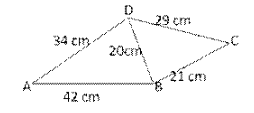Sol: Area of Q.uad. ABCD = Area of $\triangle ABD$ + Area of $\triangle DBC$

For area of $\triangle ABD$

For a = 42 cm, b = 34 cm and c = 20 cms = $\frac{a+b+c}{2}=\frac{(42+34+20)}{2}cm=48$

Then, (s – a) = 6cm, (s – b) = 14 and (s – c) = 28

Area of $\triangle ABC$ = $\sqrt{s\times (s-a)(s-b)(s-c)}$

= $\sqrt{48\times 6\times 14\times 28}cm^{2}$

= (6 * 7 * 8) $cm^{2}$ = 336 $cm^{2}$

For area of $\triangle DBC$

a = 29cm, b = 21cm, c = 20cm

s = $\frac{a+b+c}{2}=\frac{(29+21+20)}{2}cm=35cm$

(s – a) = 6cm, (s – b) = 14cm and (s – c) = 15cm

Area of $\triangle DBC$ = $\sqrt{s\times (s-a)(s-b)(s-c)}$ square units

= $\sqrt{35\times 6\times 14\times 15}cm^{2}$ = (5 * 7 * 2 * 3) $cm^{2}$ = 210 $cm^{2}$

Area of ABCD = Area of $\triangle ABD$ + Area of $\triangle DBC$ = (336 + 210) $cm^{2}$ = 546 $cm^{2}$

Q.14: Find the area of a parallelogram with base eQ.ual to 25cm and the corresponding height measuring 16.8cm.

Sol: Given:

Base = 25 cm and Height = 16.8 cm

Therefore, Area of the parallelogram = Base * Height = 25 cm * 16.8 cm =

420 $cm^{2}$

Q.15: The adjacent sides of a parallelogram are 32 cm and 24 cm. If the distance between the longer sides is 17.4 cm, find the distance between the shorter sides.

Sol: Longer side = 32 cm and Shorter side = 24 cm

Let the distance between the shorter sides be x cm.

Area of a parallelogram = Longer side * Distance between the longer sides = Shorter side * Distance between the shorter sides

or, 32 * 17.4 = 24 * x

or, x = $\frac{32\times 17.4}{24}=23.2cm$

Therefore, distance between the shorter sides = 23.2 cm

Q.16: The area of a parallelogram is 392 $m^{2}$ . If its altitude is twice the corresponding base, determine the base and the altitude.

Sol: Area of the parallelogram = 392 $m^{2}$

Let the base of the parallelogram be b m.

Given:

The height of the parallelogram is twice the base.

Therefore, Height = 2b m

Area of a parallelogram = Base * Height

i.e 392 = b * 2b

i.e 392 = $2b^{2}$

i.e $\frac{392}{2}=b^{2}$

i.e 196 = $2b^{2}$

i.e b = 14

Therefore, Base = 14 m and Altitude = 2 * Base = 2 * 14 = 28 m

Q.17: The adjacent sides of parallelogram ABCD measure 34 cm and 20 cm, and the diagonal AC measures 42 cm. Find the area of the parallelogram.

Sol: Parallelogram ABCD is made up of congruent $\triangle ABC$ and $\triangle ADC$ .

Area of triangle ABC = $\sqrt{s\times (s-a)(s-b)(s-c)}$ (Here, s is the semi perimeter)

Thus, we have:

s = $\frac{a+b+c}{2}$

s = $\frac{34+20+42}{2}$

s = 48 cm

Area of $\triangle ABC$ = $\sqrt{48\times (48-34)(48-20)(48-42)}$

$\sqrt{48\times 14\times 28\times 6}$ = 336 $cm^{2}$

Now, Area of the parallelogram = 2 *Area of $\triangle ABC$ = 2 * 336 = 672 $cm^{2}$

Q.18: Find the area of the rhombus, the lengths of whose diagonals are 30 cm and 16 cm. Also, find the perimeter of the rhombus.

Sol: Area of the rhombus = $\frac{1}{2}\times d_{1}\times d_{2}$

= $\frac{1}{2}\times 30\times 16$ = 240 $cm^{2}$

Side of the rhombus = $\frac{1}{2}\sqrt{d_{1}^{2}+d_{2}^{2}}$

= $\frac{1}{2}\sqrt{30^{2}+16^{2}}$ = $\frac{1}{2}\sqrt{1156}$ = $\frac{1}{2}\times 34$ = 17 cm

Perimeter of the rhombus = 4a = 4 * 17 = 68 cm

Q.19: The perimeter of a rhombus is 60 cm. If one of its diagonals is 18 cm long, find (i) the length of the other diagonal, and (ii) the area of the rhombus.

Sol: Perimeter of a rhombus = 4a (here, a is the side of the rhombus.)

60 = 4a

i.e a = 15 cm

(i) Given:

One of the diagonals is 18 cm long.

$d_{1}=18cm$

Thus, we have:

Side = $\frac{1}{2}\sqrt{d_{1}^{2}+d_{2}^{2}}$

i.e 15 = $\frac{1}{2}\sqrt{18^{2}+d_{2}^{2}}$

i.e $30=\sqrt{18^{2}+d_{2}^{2}}$

Squaring both sides, we get:

i.e 900 = $18^{2}+d_{2}^{2}$

i.e 900 = 324 + $d_{2}^{2}$

i.e $d_{2}^{2}$ = 576

i.e $d_{2}$ = 24 cm

Therefore, Length of the other diagonal = 24 cm

(ii) Area of the rhombus = $\frac{1}{2}d_{1}\times d_{2}$

= $\frac{1}{2}\times 18\times 24$ = 216 $cm^{2}$

Q.20: The area of a rhombus is 480 $cm^{2}$ , and one of its diagonals measures 48 cm. Find (i) the length of the other diagonal, (ii) the length of each of its sides, and (iii) its perimeter.

Sol: Area of a rhombus = $\frac{1}{2}\times d_{1}\times d_{2}$ , where $d_{1}$ and $d_{2}$ are the lengths of the diagonals.

i.e 480 = $\frac{1}{2}\times 48\times d_{2}$

i.e $d_{2}=\frac{480\times 2}{48}$

i.e $d_{2}=20cm$

Therefore, Length of the other diagonal = 20 cm

(ii) Side = $\frac{1}{2}\sqrt{d_{1}^{2}+d_{2}^{2}}$

= $\frac{1}{2}\sqrt{48^{2}+20^{2}}$ = $\frac{1}{2}\sqrt{2304+400}$

= $\frac{1}{2}\sqrt{2704}$ = $\frac{1}{2}\times 52$ = 26 cm

Therefore, length of the side of the rhombus = 26 cm

(iii) Perimeter of the rhombus = 4 * Side = 4 * 26 = 104 cm

Q.21: The parallel sides of a trapezium are 12 cm and 9 cm and the distance between them is 8 cm. Find the area of the trapezium.

Sol: Area of trapezium = $\frac{1}{2}\times (Sum\;of\;parallel\;side\;s)\times (Distance\;between\;them)$ = $\frac{1}{2}\times (12+9)\times (8)$ = 84 $cm^{2}$

Q.22: The shape of the cross section of a canal is a trapezium. If the canal is 10 m wide at the top, 6 m wide at the bottom and the area of its cross section is $640m^{2}$ , find the depth of the canal.

Sol: Area of the canal = $640m^{2}$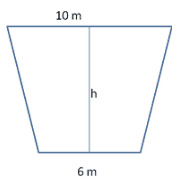Area of trapezium = $\frac{1}{2}\times (Sum\;of\;parallel\;side\;s)\times (Distance\;between\;them)$

i.e 640 = $\frac{1}{2}\times (10+6)\times h$

i.e $\frac{1280}{16}=h$

i.e h = 80 m

Therefore, the depth of the canal is 80 m.

Q.23: Find the area of a trapezium whose parallel sides are 11 m and 25 m long, and the nonparallel sides are 15 m and 13 m long.

Sol: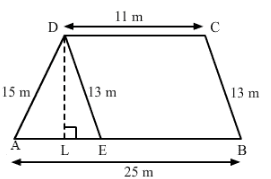Draw $DE\parallel BC$ and DL perpendicular to AB.

The opposite sides of Quadrilateral DEBC are parallel. Hence, DEBC is a parallelogram.

Therefore, DE = BC = 13 m

Also,

AE = (AB – EB) = (AB – DC) = (25 – 11) = 14 m

For $\triangle DAE$

Let:

AE = a = 14 m

DE = b = 13 m

DA = c = 15 m

Thus, we have:

$s=\frac{a+b+c}{2}$

$s=\frac{14+13+15}{2}$ = 21 m

Area of trapezium = $\frac{1}{2}\times (Sum\;of\;parallel\;side\;s)\times (Distance\;between\;them)$

= $\frac{1}{2}\times (11+25)\times 12$ = $\frac{1}{2}\times 36\times 12$ = 216 $m^{2}$

### Key Features of RS Aggarwal Class 10 Solutions Chapter 17 – Perimeter And Areas Of Plane Figures Ex 17A (17.1)

• The solutions can be referred by you whenever you feel yourself stuck anywhere.
• It is the best resource material for revision purpose.
• The RS Aggarwal Maths solutions teach different techniques to solve tough questions.
• It boosts up the confidence level for the board exam.

#### Practise This Question

The line segment AB was divided in the ratio 4:7 by taking 2 rays. The number of arcs to be made on the ray AX is: